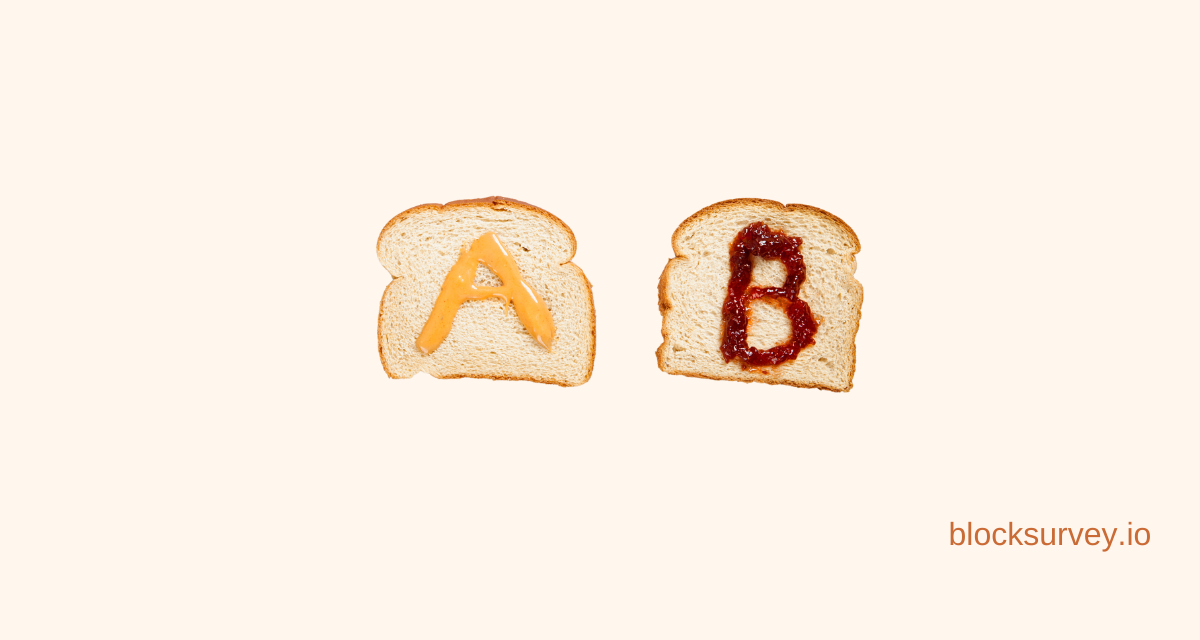# A/B Testing calculator for Statistical SignificanceAug 10, 2022 · 4 mins readIf you are testing one of your new hair oils that prevent hair fall with a different set of people, Group 1, Group 2, where only Group 1 received the hair oil and group 2 didn’t.

After two weeks of working on the results, you find significant results for both the groups for their hair fall. So what made this vast difference in the given period? Was it your hair oil, or was it by chance?

Statistical significance calculator is there for your relief!

## Okay now, what is Statistical Significance?

Statistical significance is a deciding factor in a relationship between one or more variables that are not caused by chance.

• Statistical significance is beneficial in providing evidence concerned with the plausibility of the null hypothesis, which states that there is nothing more than an arbitrary chance at work in the data.
• Statistical hypothesis testing is used to find whether the result of a data is statistically significant.
• A statistical significance calculator is used to find whether the derived data is statistically significant or derived by chance.

## What is the p-value of Statistical Significance?

It is the measure of the probability that a difference could have been due to random chance.

If the p-value is lesser, the statistical significance would be higher than the difference. It is calculated using spreadsheets, p-value tables, or any statistical software.

If a p-value, calculated from a statistical test, is 0.354, it can be 3.54% random by chance. In terms of maths, it can be calculated by integral calculation.

A null hypothesis states that there lies no relationship between the two things you are trying to equate or compare. But the alternative hypothesis states that it tries to prove a relationship that exists between the thing you are comparing.

In simple terms, it is the probability that the null hypothesis can be true. And it denotes the stronger relationship between two different variables.

If P-value falls below the level of significance, the result is said to be statistically significant.

## What is an A/b test calculator?

It is a research methodology that is also known as split testing. In a survey, it helps in making data-driven decisions rather than making impulsive decisions.

It gives new direction to your work by providing confident answers through the survey you conduct. A/B testing provides you with low-risk modifications and helps you understand the pain points of your target audience. It also helps in decreasing the bounce rate.

Before using A/B testing calculators, research well to provide the users with the right variations. Create and formulate well-researched hypotheses before running the test.

Here is a step-by-step guide to starting with A/B testing,

##Img<a/b-testing>

1. Research
2. Formulate hypothesis
3. Create different variations
4. Run a test
5. Analyze the results

## Benefits of statistical significance:

Statistical significance helps in determining whether the data supports or doesn’t support the null hypothesis. It helps in identifying whether the results are due to chance or due to some factor. It gives the strength of the evidence. The statistical significance calculator is also said to have more accuracy.

## Conclusion:

Statistical significance calculators are essential in decision-making. A statistically significant data creates a positive impact on the outlook of your website/application and paves the way to a better conversion rate. For analyzing and to get deeper insights, it is essential to have data that is statistically significant. Use tools that are relevant to get statistically significant data and to control errors in the null hypothesis. And if you overload the survey with questions, you are more likely to get irrelevant results.

Also try: Calculator Templates

Get future posts from BlockSurvey

### Like what you see? Share with a friend.### Vimala Balamurugan

Vimala heads the Content and SEO Team at BlockSurvey. She is the curator of all the content that BlockSurvey puts out into the public domain. Blogging, music, and exploring new places around is how she spends most of her leisure time.